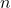# Comparing ratio estimators based on systematic samples

The purpose of this study is to evaluate competitive ratio estimators, and introduce
a new approach to estimation when a systematic sample of sizewith
a random start is used. The competitive ratio estimators are the mean of ratios,
ratio of means, and the conditional best linear unbiased estimator. These
estimators are used to measure the population proportion of the total of a variablewith respect to another variable,. A new approach is suggested, a
bootstrap estimate using a non-linear additive regression technique, in which a
Monte-Carlo simulation is done using the predicted values from the fitted model
to find estimates for the variances. This new approach has yielded small mean
square errors.

Fulltext